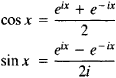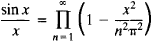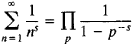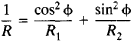# Euler's Formula

(redirected from Euler formulas)

## Euler's formula

[′ȯi·lərz ‚fȯr·myə·lə]
(mathematics)
The formula e ix = cos x + i sin x, where i = √(-1).
McGraw-Hill Dictionary of Scientific & Technical Terms, 6E, Copyright © 2003 by The McGraw-Hill Companies, Inc.
The following article is from The Great Soviet Encyclopedia (1979). It might be outdated or ideologically biased.

## Euler’s Formula

any of several important formulas established by L. Euler.

(1) A formula giving the relation between the exponential function and trigonometric functions (1743):

eix = cos x + i sin x

Also known as Euler’s formulas are the equations(2) A formula giving the expansion of the function sin x in an infinite product (1740):(3) The formulawhere s = 1,2,... and p runs over all prime numbers.

(4) The formula

(a2 + b2 + c2 + d2)(p2 + q2 + r2 + s2) = x2 + y2 + z2 + t2

where

x = ap + bq + cr + ds

y = aqbp ± csdr

z = arbscp ± dq

t = as ± brcqdp

(5) The formula (1760)Also known as the equation of Euler, it gives an expression for the curvature 1/R of a normal section of a surface in terms of the surface’s principal curvatures 1/R1 and 1/R2 and the angle φ between one of the principal directions and the given direction.

Other well-known formulas associated with Euler include the Euler-Maclaurin summation formula and the Euler-Fourier formulas for the coefficients of expansions of functions in trigonometric series.